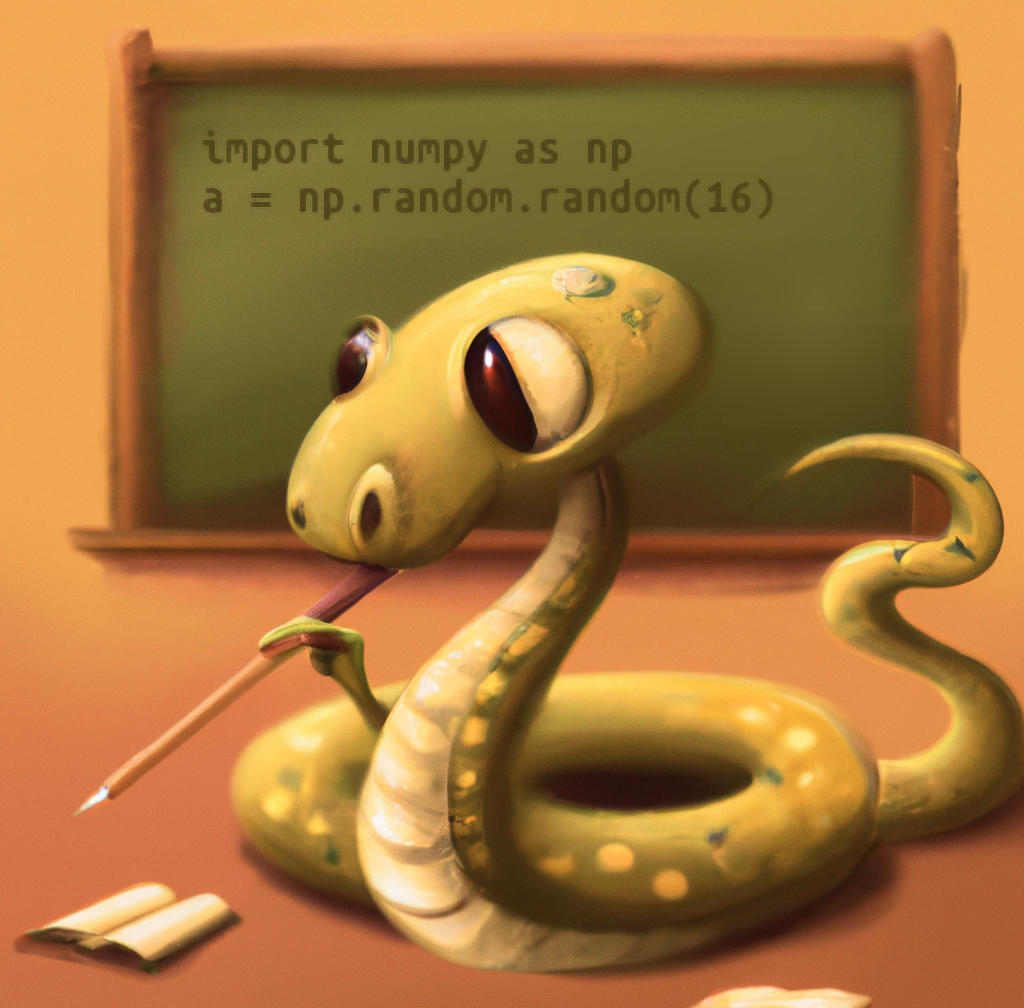# Introduction to data science with Python

In this course, you will learn the basic principles of data science with Python. That is, you will learn how to work with the most important Python libraries for numerical computing, to work with different kinds of data (arrays, column-based data, and time series), to create plots, and to perform statistical analyses.Figure 1. Your friendly Python teacher

## Installing required packages

Before you get started, you need to install several libraries that you will use (either directly or indirectly) during the course. You can do this with `pip`:

``````pip install numpy scipy matplotlib seaborn statsmodels pandas pingouin python-datamatrix prettytable fastnumbers openpyxl
``````

Or with `conda`:

``````conda install numpy scipy matplotlib seaborn statsmodels pandas pingouin datamatrix prettytable fastnumbers openpyxl
``````

## Course overview

Once you're all set, let's dive into the chapters! Each chapter builds on the previous chapters, so it is recommended to follow the chapters in order!

1. Introduction to data science with Python (this chapter)
2. Working with NumPy arrays
3. Working with tabular data using DataMatrix
4. Plotting with Seaborn and Maplotlib
5. Statistics with SciPy, Statsmodels, and Pingouin
6. Analyzing time series with DataMatrix

You're done with this section!